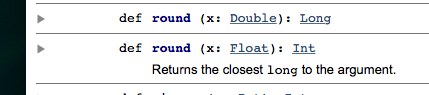# How to round to Int

scala.Math apparently has two methods named round. But the documentation looks wrong to me.
Am I confused?
The method declared to return type Int is documented to return the closest Long.How can I specify which method I want to call if they only distinguish by return value? In the following local function, scale is a value declared a few lines up as Double. My intention is to round to the nearest integer, but IntelliJ tells me that my function declared as returning Int does not conform to the type Long. What’s the correct way to fix my problem?

``````       def interpolate(lower: Int, upper: Int): Int = {
min(255, lower + round((upper - lower) * scale))
}``````

Hello,

The methods are distinguished by argument types. Give it a Float, and you
will get a Double:

scala> Math.round(2.5)
res1: Long = 3

scala> Math.round(2.5f)
res2: Int = 3

scala> Math.round(2.5.floatValue)
res4: Int = 3

`` Best, Oliver``
1 Like

Sorry, obviously, I meant: “Give it a Float, and you will get an Int”

The scaladoc is clearly wrong though.

EDIT: However, that was already fixed a long time ago: https://github.com/scala/scala/commit/1994a2d901e1ca948a53b3d00df9017782c47b5c

1 Like

Thanks for the push into the right direction. But I’m still not quite seeing how to make
the result of my calculation be an Int. What should I change in the following function so that the function really is (Int,Int,Double)=>Int ?

``````       def interpolate(lower: Int, upper: Int, scale:Double ): Int = {
max(0,min(255, lower + round((upper - lower) * scale)))
}``````

How do you want to handle overflow?

I’ll just throw out that the operations you are doing, like min, max, and round, are also methods on Double and Float (Int and Long have min and max). So you could consider writing your method in the following way.

``````0L max 255L min lower + ((upper - lower) * scale).round
``````

Note that I had to make the bounds be Longs because min and max require the same types.

As for getting out an Int, it has already been asked how you want to handle overflow because Double can hold larger whole numbers than Int can. The simplest approach is the toInt method, which just ignores overflows, and the value wraps around. However, if you call toInt after your min and max have occurred, you know that the value is between 0 and 255, so there is no possibility that part of the expression can overflow and toInt is safe.

``````(0L max 255L min lower + ((upper - lower) * scale).round).toInt
``````

Granted, the round can still have problems if the Double expression is larger than Long.MaxValue. The round method simply truncates, so 1e100.round is Long.MaxValue.

Hello,

You could simply do:

def interpolate(lower: Int, upper: Int, scale:Double ): Int = {
max(0,min(255, lower + round(((upper - lower) * scale).floatValue)))
}

or

def interpolate(lower: Int, upper: Int, scale:Double ): Int = {
max(0,min(255, lower + round((upper - lower) * scale))).intValue
}

BTW, why do you need an Int?

`` Best, Oliver``

overflow? Will I have overflow at all, since I’m taking max with 0 and min with 255?

Why do I need Int? It is because I’m using another class which I didn’t write, and I am forbidden to change, that class needs its fields as Int’s.

But even that is curios to me. The class that I’m using is a Color class which has red, green, and blue fields which are Int between 0 and 255. Is Int really the best numerical value for values x: 0 <= x <= 255 ? Even if that’s not appropriate, I can’t change it. But I am curious.

Considering that Math.round truncates large Float/Double values to
Int.Max/Long.max, and it looks like your code handles overflow well.

If Color takes Ints, of course you provide Ints.

Is Int the most efficient? Short also covers 0 to 255. A decade or two
ago, most hardware architecture was 32 bit, so Float/Int were likely the
most efficient types. Now, most architectures are 64 bit, so now
Double/Long are probably the most efficient, but code remains that uses
Float/Int.